# NumPy - 简介

NumPy 是一个 Python 包。 它代表 “Numeric Python”。 它是一个由多维数组对象和用于处理数组的例程集合组成的库。

Numeric，即 NumPy 的前身，是由 Jim Hugunin 开发的。 也开发了另一个包 Numarray ，它拥有一些额外的功能。 2005年，Travis Oliphant 通过将 Numarray 的功能集成到 Numeric 包中来创建 NumPy 包。 这个开源项目有很多贡献者。

## NumPy 操作

• 数组的算数和逻辑运算。

• 傅立叶变换和用于图形操作的例程。

• 与线性代数有关的操作。 NumPy 拥有线性代数和随机数生成的内置函数。

## NumPy – MatLab 的替代之一

NumPy 通常与 SciPy（Scientific Python）和 Matplotlib（绘图库）一起使用。 这种组合广泛用于替代 MatLab，是一个流行的技术计算平台。 但是，Python 作为 MatLab 的替代方案，现在被视为一种更加现代和完整的编程语言。

NumPy 是开源的，这是它的一个额外的优势。

# NumPy - 环境

### 在线尝试

```import numpy as np
a =  'hello world'  print a```

`pip install numpy`

## Windows

Anaconda (from www.continuum.io) 是一个带有 SciPy 技术栈的免费 Python 发行版。 它也可用于 Linux 和 Mac.

Canopy (www.enthought.com/products/canopy/) 是可用的免费和商业发行版，带有完整的 SciPy 技术栈，可用于 Windows, Linux and Mac。

Python (x,y): 是个免费的 Python 发行版，带有 SciPy 技术栈和 Spyder IDE，可用于 Windows。 (从这里下载：www.python-xy.github.io/)

## Linux

Linux 发行版的相应软件包管理器可用于安装一个或多个 SciPy 技术栈中的软件包。

## 对于 Ubuntu

```sudo apt-get install python-numpy
python-scipy python-matplotlibipythonipythonnotebook python-pandas
python-sympy python-nose```

## 对于 Fedora

```sudo yum install numpyscipy python-matplotlibipython
python-pandas sympy python-nose atlas-devel```

## 从源码构建

GNU gcc（4.2及以上）C 编译器必须可用。

`Python setup.py install`

```Traceback (most recent call last):
File "<pyshell#0>", line 1, in <module>
import numpy
ImportError: No module named 'numpy'```

# NumPy - Ndarray 对象

NumPy 中定义的最重要的对象是称为 `ndarray` 的 N 维数组类型。 它描述相同类型的元素集合。 可以使用基于零的索引访问集合中的项目。

`ndarray`中的每个元素在内存中使用相同大小的块。 `ndarray`中的每个元素是数据类型对象的对象（称为 `dtype`）。

`ndarray`对象提取的任何元素（通过切片）由一个数组标量类型的 Python 对象表示。 下图显示了`ndarray`，数据类型对象（`dtype`）和数组标量类型之间的关系。Ndarray

`ndarray`类的实例可以通过本教程后面描述的不同的数组创建例程来构造。 基本的`ndarray`是使用 NumPy 中的数组函数创建的，如下所示：

`numpy.array`

`numpy.array(object, dtype = None, copy = True, order = None, subok = False, ndmin = 0)`

1.`object` 任何暴露数组接口方法的对象都会返回一个数组或任何（嵌套）序列。
2.`dtype` 数组的所需数据类型，可选。
3.`copy` 可选，默认为`true`，对象是否被复制。
4.`order` `C`（按行）、`F`（按列）或`A`（任意，默认）。
5.`subok` 默认情况下，返回的数组被强制为基类数组。 如果为`true`，则返回子类。
6.`ndimin` 指定返回数组的最小维数。

## 示例 1

```import numpy as np
a = np.array([1,2,3])  print a```

`[1, 2, 3]`

## 示例 2

```# 多于一个维度  import numpy as np
a = np.array([[1,  2],  [3,  4]])  print a```

```[[1, 2]
[3, 4]]```

## 示例 3

```# 最小维度  import numpy as np
a = np.array([1,  2,  3,4,5], ndmin =  2)  print a```

`[[1, 2, 3, 4, 5]]`

## 示例 4

```# dtype 参数  import numpy as np
a = np.array([1,  2,  3], dtype = complex)  print a```

`[ 1.+0.j,  2.+0.j,  3.+0.j]`

**ndarray ** 对象由计算机内存中的一维连续区域组成，带有将每个元素映射到内存块中某个位置的索引方案。 内存块以按行（C 风格）或按列（FORTRAN 或 MatLab 风格）的方式保存元素。

# NumPy - 数据类型

NumPy 支持比 Python 更多种类的数值类型。 下表显示了 NumPy 中定义的不同标量数据类型。

1.`bool_` 存储为一个字节的布尔值（真或假）
2.`int_` 默认整数，相当于 C 的`long`，通常为`int32``int64`
3.`intc` 相当于 C 的`int`，通常为`int32``int64`
4.`intp` 用于索引的整数，相当于 C 的`size_t`，通常为`int32``int64`
5.`int8` 字节（-128 ~ 127）
6.`int16` 16 位整数（-32768 ~ 32767）
7.`int32` 32 位整数（-2147483648 ~ 2147483647）
8.`int64` 64 位整数（-9223372036854775808 ~ 9223372036854775807）
9.`uint8` 8 位无符号整数（0 ~ 255）
10.`uint16` 16 位无符号整数（0 ~ 65535）
11.`uint32` 32 位无符号整数（0 ~ 4294967295）
12.`uint64` 64 位无符号整数（0 ~ 18446744073709551615）
13.`float_` `float64`的简写
14.`float16` 半精度浮点：符号位，5 位指数，10 位尾数
15.`float32` 单精度浮点：符号位，8 位指数，23 位尾数
16.`float64` 双精度浮点：符号位，11 位指数，52 位尾数
17.`complex_` `complex128`的简写
18.`complex64` 复数，由两个 32 位浮点表示（实部和虚部）
19.`complex128` 复数，由两个 64 位浮点表示（实部和虚部）

NumPy 数字类型是`dtype`（数据类型）对象的实例，每个对象具有唯一的特征。 这些类型可以是`np.bool_``np.float32`等。

## 数据类型对象 (`dtype`)

• 数据类型（整数、浮点或者 Python 对象）

• 数据大小

• 字节序（小端或大端）

• 在结构化类型的情况下，字段的名称，每个字段的数据类型，和每个字段占用的内存块部分。

• 如果数据类型是子序列，它的形状和数据类型。

`dtype`可由一下语法构造：

`numpy.dtype(object, align, copy)`

• `Object`：被转换为数据类型的对象。

• `Align`：如果为`true`，则向字段添加间隔，使其类似 C 的结构体。

• `Copy` ? 生成`dtype`对象的新副本，如果为`flase`，结果是内建数据类型对象的引用。

### 示例 1

```# 使用数组标量类型  import numpy as np
dt = np.dtype(np.int32)  print dt```

`int32`

### 示例 2

```#int8，int16，int32，int64 可替换为等价的字符串 'i1'，'i2'，'i4'，以及其他。
import numpy as np

dt = np.dtype('i4')  print dt```

`int32`

### 示例 3

```# 使用端记号  import numpy as np
dt = np.dtype('>i4')  print dt```

`>i4`

### 示例 4

```# 首先创建结构化数据类型。  import numpy as np
dt = np.dtype([('age',np.int8)])  print dt```

`[('age', 'i1')]`

### 示例 5

```# 现在将其应用于 ndarray 对象  import numpy as np

dt = np.dtype([('age',np.int8)]) a = np.array([(10,),(20,),(30,)], dtype = dt)  print a```

`[(10,) (20,) (30,)]`

### 示例 6

```# 文件名称可用于访问 age 列的内容  import numpy as np

dt = np.dtype([('age',np.int8)]) a = np.array([(10,),(20,),(30,)], dtype = dt)  print a['age']```

`[10 20 30]`

### 示例 7

```import numpy as np
student = np.dtype([('name','S20'),  ('age',  'i1'),  ('marks',  'f4')])  print student```

`[('name', 'S20'), ('age', 'i1'), ('marks', '<f4')])`

### 示例 8

```import numpy as np

student = np.dtype([('name','S20'),  ('age',  'i1'),  ('marks',  'f4')]) a = np.array([('abc',  21,  50),('xyz',  18,  75)], dtype = student)  print a```

`[('abc', 21, 50.0), ('xyz', 18, 75.0)]`

• `'b'`：布尔值

• `'i'`：符号整数

• `'u'`：无符号整数

• `'f'`：浮点

• `'c'`：复数浮点

• `'m'`：时间间隔

• `'M'`：日期时间

• `'O'`：Python 对象

• `'S', 'a'`：字节串

• `'U'`：Unicode

• `'V'`：原始数据（`void`

# NumPy - 数组属性

## `ndarray.shape`

### 示例 1

```import numpy as np
a = np.array([[1,2,3],[4,5,6]])  print a.shape```

`(2, 3)`

### 示例 2

```# 这会调整数组大小  import numpy as np

a = np.array([[1,2,3],[4,5,6]]) a.shape =  (3,2)  print a```

```[[1, 2]
[3, 4]
[5, 6]]```

### 示例 3

NumPy 也提供了`reshape`函数来调整数组大小。

```import numpy as np
a = np.array([[1,2,3],[4,5,6]]) b = a.reshape(3,2)  print b```

```[[1, 2]
[3, 4]
[5, 6]]```

## `ndarray.ndim`

### 示例 1

```# 等间隔数字的数组  import numpy as np
a = np.arange(24)  print a```

`[0 1  2  3  4  5  6  7  8  9  10  11  12  13  14  15  16 17 18 19 20 21 22 23]`

### 示例 2

```# 一维数组  import numpy as np
a = np.arange(24) a.ndim
# 现在调整其大小b = a.reshape(2,4,3)  print b
# b 现在拥有三个维度```

```[[[ 0,  1,  2]
[ 3,  4,  5]
[ 6,  7,  8]
[ 9, 10, 11]]
[[12, 13, 14]
[15, 16, 17]
[18, 19, 20]
[21, 22, 23]]]```

## `numpy.itemsize`

### 示例 1

```# 数组的 dtype 为 int8（一个字节）
import numpy as np
x = np.array([1,2,3,4,5], dtype = np.int8)  print x.itemsize```

`1`

### 示例 2

```# 数组的 dtype 现在为 float32（四个字节）
import numpy as np
x = np.array([1,2,3,4,5], dtype = np.float32)  print x.itemsize```

`4`

## `numpy.flags`

`ndarray`对象拥有以下属性。这个函数返回了它们的当前值。

1.`C_CONTIGUOUS (C)` 数组位于单一的、C 风格的连续区段内
2.`F_CONTIGUOUS (F)` 数组位于单一的、Fortran 风格的连续区段内
3.`OWNDATA (O)` 数组的内存从其它对象处借用
4.`WRITEABLE (W)` 数据区域可写入。 将它设置为`flase`会锁定数据，使其只读
5.`ALIGNED (A)` 数据和任何元素会为硬件适当对齐
6.`UPDATEIFCOPY (U)` 这个数组是另一数组的副本。当这个数组释放时，源数组会由这个数组中的元素更新

### 示例

```import numpy as np
x = np.array([1,2,3,4,5])  print x.flags```

`C_CONTIGUOUS : True F_CONTIGUOUS : True OWNDATA : True WRITEABLE : True ALIGNED : True UPDATEIFCOPY : False`

# NumPy - 数组创建例程

## `numpy.empty`

`numpy.empty(shape, dtype = float, order = 'C')`

1.`Shape` 空数组的形状，整数或整数元组
2.`Dtype` 所需的输出数组类型，可选
3.`Order` `'C'`为按行的 C 风格数组，`'F'`为按列的 Fortran 风格数组

### 示例

```import numpy as np
x = np.empty([3,2], dtype =  int)  print x```

```[[22649312    1701344351]
[1818321759  1885959276]
[16779776    156368896]]```

## `numpy.zeros`

`numpy.zeros(shape, dtype = float, order = 'C')`

1.`Shape` 空数组的形状，整数或整数元组
2.`Dtype` 所需的输出数组类型，可选
3.`Order` `'C'`为按行的 C 风格数组，`'F'`为按列的 Fortran 风格数组

### 示例 1

```# 含有 5 个 0 的数组，默认类型为 float  import numpy as np
x = np.zeros(5)  print x```

`[ 0.  0.  0.  0.  0.]`

### 示例 2

```import numpy as np
x = np.zeros((5,), dtype = np.int)  print x```

`[0  0  0  0  0]`

### 示例 3

```# 自定义类型 import numpy as np
x = np.zeros((2,2), dtype =  [('x',  'i4'),  ('y',  'i4')])  print x```

```[[(0,0)(0,0)]
[(0,0)(0,0)]]```

## `numpy.ones`

`numpy.ones(shape, dtype = None, order = 'C')`

1.`Shape` 空数组的形状，整数或整数元组
2.`Dtype` 所需的输出数组类型，可选
3.`Order` `'C'`为按行的 C 风格数组，`'F'`为按列的 Fortran 风格数组

### 示例 1

```# 含有 5 个 1 的数组，默认类型为 float  import numpy as np
x = np.ones(5)  print x```

`[ 1.  1.  1.  1.  1.]`

### 示例 2

```import numpy as np
x = np.ones([2,2], dtype =  int)  print x```

```[[1  1]
[1  1]]```

# NumPy - 来自现有数据的数组

## `numpy.asarray`

`numpy.asarray(a, dtype = None, order = None)`

1.`a` 任意形式的输入参数，比如列表、列表的元组、元组、元组的元组、元组的列表
2.`dtype` 通常，输入数据的类型会应用到返回的`ndarray`
3.`order` `'C'`为按行的 C 风格数组，`'F'`为按列的 Fortran 风格数组

### 示例 1

```# 将列表转换为 ndarray import numpy as np

x =  [1,2,3] a = np.asarray(x)  print a```

`[1  2  3]`

### 示例 2

```# 设置了 dtype  import numpy as np

x =  [1,2,3] a = np.asarray(x, dtype =  float)  print a```

`[ 1.  2.  3.]`

### 示例 3

```# 来自元组的 ndarray  import numpy as np

x =  (1,2,3) a = np.asarray(x)  print a```

`[1  2  3]`

### 示例 4

```# 来自元组列表的 ndarrayimport numpy as np

x =  [(1,2,3),(4,5)] a = np.asarray(x)  print a```

`[(1, 2, 3) (4, 5)]`

## `numpy.frombuffer`

`numpy.frombuffer(buffer, dtype = float, count = -1, offset = 0)`

1.`buffer` 任何暴露缓冲区借口的对象
2.`dtype` 返回数组的数据类型，默认为`float`
3.`count` 需要读取的数据数量，默认为`-1`，读取所有数据
4.`offset` 需要读取的起始位置，默认为`0`

### 示例

```import numpy as np
s =  'Hello World' a = np.frombuffer(s, dtype =  'S1')  print a```

`['H'  'e'  'l'  'l'  'o'  ' '  'W'  'o'  'r'  'l'  'd']`

## `numpy.fromiter`

`numpy.fromiter(iterable, dtype, count = -1)`

1.`iterable` 任何可迭代对象
2.`dtype` 返回数组的数据类型
3.`count` 需要读取的数据数量，默认为`-1`，读取所有数据

### 示例 1

```# 使用 range 函数创建列表对象  import numpy as np
list = range(5)  print list```

`[0,  1,  2,  3,  4]`

### 示例 2

```# 从列表中获得迭代器  import numpy as np
list = range(5) it = iter(list)  # 使用迭代器创建 ndarray x = np.fromiter(it, dtype =  float)  print x```

`[0.   1.   2.   3.   4.]`

# NumPy - 来自数值范围的数组

## `numpy.arange`

`numpy.arange(start, stop, step, dtype)`

1.`start` 范围的起始值，默认为`0`
2.`stop` 范围的终止值（不包含）
3.`step` 两个值的间隔，默认为`1`
4.`dtype` 返回`ndarray`的数据类型，如果没有提供，则会使用输入数据的类型。

### 示例 1

```import numpy as np
x = np.arange(5)  print x```

`[0  1  2  3  4]`

### 示例 2

`import numpy as np# 设置了 dtypex = np.arange(5, dtype =  float)  print x`

`[0.  1.  2.  3.  4.]`

### 示例 3

```# 设置了起始值和终止值参数  import numpy as np
x = np.arange(10,20,2)  print x```

`[10  12  14  16  18]`

## `numpy.linspace`

`numpy.linspace(start, stop, num, endpoint, retstep, dtype)`

1.`start` 序列的起始值
2.`stop` 序列的终止值，如果`endpoint``true`，该值包含于序列中
3.`num` 要生成的等间隔样例数量，默认为`50`
4.`endpoint` 序列中是否包含`stop`值，默认为`ture`
5.`retstep` 如果为`true`，返回样例，以及连续数字之间的步长
6.`dtype` 输出`ndarray`的数据类型

### 示例 1

```import numpy as np
x = np.linspace(10,20,5)  print x```

`[10.   12.5   15.   17.5  20.]`

### 示例 2

```# 将 endpoint 设为 falseimport numpy as np
x = np.linspace(10,20,  5, endpoint =  False)  print x```

`[10.   12.   14.   16.   18.]`

### 示例 3

```# 输出 retstep 值  import numpy as np

x = np.linspace(1,2,5, retstep =  True)  print x# 这里的 retstep 为 0.25```

`(array([ 1.  ,  1.25,  1.5 ,  1.75,  2.  ]), 0.25)`

## `numpy.logspace`

`numpy.logscale(start, stop, num, endpoint, base, dtype)`

`logspace`函数的输出由以下参数决定：

1.`start` 起始值是`base ** start`
2.`stop` 终止值是`base ** stop`
3.`num` 范围内的数值数量，默认为`50`
4.`endpoint` 如果为`true`，终止值包含在输出数组当中
5.`base` 对数空间的底数，默认为`10`
6.`dtype` 输出数组的数据类型，如果没有提供，则取决于其它参数

### 示例 1

```import numpy as np
# 默认底数是 10a = np.logspace(1.0,  2.0, num =  10)  print a```

```[ 10.           12.91549665     16.68100537      21.5443469  27.82559402
35.93813664   46.41588834     59.94842503      77.42636827    100.    ]```

### 示例 2

```# 将对数空间的底数设置为 2  import numpy as np
a = np.logspace(1,10,num =  10,  base  =  2)  print a```

`[ 2.     4.     8.    16.    32.    64.   128.   256.    512.   1024.]`

# NumPy - 切片和索引

`ndarray`对象的内容可以通过索引或切片来访问和修改，就像 Python 的内置容器对象一样。

## 示例 1

```import numpy as np
a = np.arange(10)s = slice(2,7,2)  print a[s]```

`[2  4  6]`

## 示例 2

```import numpy as np
a = np.arange(10)b = a[2:7:2]  print b```

`[2  4  6]`

## 示例 3

```# 对单个元素进行切片  import numpy as np

a = np.arange(10)b = a  print b```

`5`

## 示例 4

```# 对始于索引的元素进行切片  import numpy as np
a = np.arange(10)  print a[2:]```

`[2  3  4  5  6  7  8  9]`

## 示例 5

```# 对索引之间的元素进行切片  import numpy as np
a = np.arange(10)  print a[2:5]```

`[2  3  4]`

## 示例 6

```import numpy as np
a = np.array([[1,2,3],[3,4,5],[4,5,6]])  print a# 对始于索引的元素进行切片  print  '现在我们从索引 a[1:] 开始对数组切片'  print a[1:]```

```[[1 2 3]
[3 4 5]
[4 5 6]]

[[3 4 5]
[4 5 6]]```

## 示例 7

```# 最开始的数组  import numpy as np
a = np.array([[1,2,3],[3,4,5],[4,5,6]])  print  '我们的数组是：'  print aprint  '\n'  # 这会返回第二列元素的数组：  print  '第二列的元素是：'  print a[...,1]  print  '\n'  # 现在我们从第二行切片所有元素：  print  '第二行的元素是：'  print a[1,...]  print  '\n'  # 现在我们从第二列向后切片所有元素：print  '第二列及其剩余元素是：'  print a[...,1:]```

```我们的数组是：[[1 2 3]
[3 4 5]
[4 5 6]]第二列的元素是：[2 4 5]第二行的元素是：[3 4 5]第二列及其剩余元素是：[[2 3]
[4 5]
[5 6]]```

# NumPy - 高级索引

## 整数索引

### 示例 1

```import numpy as np

x = np.array([[1,  2],  [3,  4],  [5,  6]]) y = x[[0,1,2],  [0,1,0]]  print y```

`[1  4  5]`

### 示例 2

```import numpy as np
x = np.array([[  0,  1,  2],[  3,  4,  5],[  6,  7,  8],[  9,  10,  11]])  print  '我们的数组是：'  print x
print  '\n' rows = np.array([[0,0],[3,3]]) cols = np.array([[0,2],[0,2]]) y = x[rows,cols]  print  '这个数组的每个角处的元素是：'  print y```

```我们的数组是：
[[ 0  1  2]
[ 3  4  5]
[ 6  7  8]
[ 9 10 11]]这个数组的每个角处的元素是：
[[ 0  2]
[ 9 11]]```

### 示例 3

```import numpy as np
x = np.array([[  0,  1,  2],[  3,  4,  5],[  6,  7,  8],[  9,  10,  11]])  print  '我们的数组是：'  print x
print  '\n'  # 切片z = x[1:4,1:3]  print  '切片之后，我们的数组变为：'  print z
print  '\n'  # 对列使用高级索引 y = x[1:4,[1,2]] print  '对列使用高级索引来切片：'  print y```

```我们的数组是：[[ 0  1  2]
[ 3  4  5]
[ 6  7  8]
[ 9 10 11]]切片之后，我们的数组变为：[[ 4  5]
[ 7  8]
[10 11]]对列使用高级索引来切片：[[ 4  5]
[ 7  8]
[10 11]]```

## 布尔索引

### 示例 1

```import numpy as np
x = np.array([[  0,  1,  2],[  3,  4,  5],[  6,  7,  8],[  9,  10,  11]])  print  '我们的数组是：'  print x
print  '\n'  # 现在我们会打印出大于 5 的元素  print  '大于 5 的元素是：'  print x[x >  5]```

```我们的数组是：[[ 0  1  2]
[ 3  4  5]
[ 6  7  8]
[ 9 10 11]] 大于 5 的元素是：[ 6  7  8  9 10 11]```

### 示例 2

```import numpy as np
a = np.array([np.nan,  1,2,np.nan,3,4,5])  print a[~np.isnan(a)]```

`[ 1.   2.   3.   4.   5.]`

### 示例 3

```import numpy as np
a = np.array([1,  2+6j,  5,  3.5+5j])  print a[np.iscomplex(a)]```

`[2.0+6.j  3.5+5.j]`

# NumPy - 广播

## 示例 1

```import numpy as np

a = np.array([1,2,3,4]) b = np.array([10,20,30,40]) c = a * b
print c```

`[10   40   90   160]`

• `ndim`较小的数组会在前面追加一个长度为 1 的维度。

• 输出数组的每个维度的大小是输入数组该维度大小的最大值。

• 如果输入在每个维度中的大小与输出大小匹配，或其值正好为 1，则在计算中可它。

• 如果输入的某个维度大小为 1，则该维度中的第一个数据元素将用于该维度的所有计算。

• 数组拥有相同形状。

• 数组拥有相同的维数，每个维度拥有相同长度，或者长度为 1。

• 数组拥有极少的维度，可以在其前面追加长度为 1 的维度，使上述条件成立。

## 示例 2

```import numpy as np
a = np.array([[0.0,0.0,0.0],[10.0,10.0,10.0],[20.0,20.0,20.0],[30.0,30.0,30.0]]) b = np.array([1.0,2.0,3.0])  print  '第一个数组：'  print a
print  '\n'  print  '第二个数组：'  print b
print  '\n'  print  '第一个数组加第二个数组：'  print a + b```

```第一个数组：[[ 0. 0. 0.]
[ 10. 10. 10.]
[ 20. 20. 20.]
[ 30. 30. 30.]]第二个数组：[ 1. 2. 3.]第一个数组加第二个数组：[[ 1. 2. 3.]
[ 11. 12. 13.]
[ 21. 22. 23.]
[ 31. 32. 33.]]```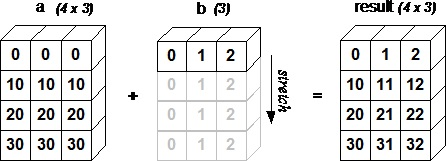array

# NumPy - 数组上的迭代

NumPy 包包含一个迭代器对象`numpy.nditer`。 它是一个有效的多维迭代器对象，可以用于在数组上进行迭代。 数组的每个元素可使用 Python 的标准`Iterator`接口来访问。

### 示例 1

```import numpy as np
a = np.arange(0,60,5) a = a.reshape(3,4)  print  '原始数组是：'  print a print  '\n'  print  '修改后的数组是：'  for x in np.nditer(a):
print x,```

```原始数组是：[[ 0 5 10 15]
[20 25 30 35]
[40 45 50 55]]修改后的数组是：0 5 10 15 20 25 30 35 40 45 50 55```

### 示例 2

```import numpy as np
a = np.arange(0,60,5) a = a.reshape(3,4)  print  '原始数组是：'  print a
print  '\n'  print  '原始数组的转置是：' b = a.T
print b
print  '\n'  print  '修改后的数组是：'  for x in np.nditer(b):
print x,```

```原始数组是：[[ 0 5 10 15]
[20 25 30 35]
[40 45 50 55]]原始数组的转置是：[[ 0 20 40]
[ 5 25 45]
[10 30 50]
[15 35 55]]修改后的数组是：0 5 10 15 20 25 30 35 40 45 50 55```

## 迭代顺序

### 示例 1

```import numpy as np
a = np.arange(0,60,5) a = a.reshape(3,4)  print  '原始数组是：'  print a print  '\n'  print  '原始数组的转置是：' b = a.T
print b
print  '\n'  print  '以 C 风格顺序排序：' c = b.copy(order='C')  print c for x in np.nditer(c):
print x,  print  '\n'  print  '以 F 风格顺序排序：' c = b.copy(order='F')  print c
for x in np.nditer(c):
print x,```

```原始数组是：[[ 0 5 10 15]
[20 25 30 35]
[40 45 50 55]]原始数组的转置是：[[ 0 20 40]
[ 5 25 45]
[10 30 50]
[15 35 55]]以 C 风格顺序排序：[[ 0 20 40]
[ 5 25 45]
[10 30 50]
[15 35 55]]0 20 40 5 25 45 10 30 50 15 35 55以 F 风格顺序排序：[[ 0 20 40]
[ 5 25 45]
[10 30 50]
[15 35 55]]0 5 10 15 20 25 30 35 40 45 50 55```

### 示例 2

```import numpy as np
a = np.arange(0,60,5) a = a.reshape(3,4)  print  '原始数组是：'  print a
print  '\n'  print  '以 C 风格顺序排序：'  for x in np.nditer(a, order =  'C'):
print x,  print  '\n'  print  '以 F 风格顺序排序：'  for x in np.nditer(a, order =  'F'):
print x,```

```原始数组是：[[ 0 5 10 15]
[20 25 30 35]
[40 45 50 55]]以 C 风格顺序排序：0 5 10 15 20 25 30 35 40 45 50 55以 F 风格顺序排序：0 20 40 5 25 45 10 30 50 15 35 55```

## 修改数组的值

`nditer`对象有另一个可选参数`op_flags`。 其默认值为只读，但可以设置为读写或只写模式。 这将允许使用此迭代器修改数组元素。

### 示例

```import numpy as np
a = np.arange(0,60,5) a = a.reshape(3,4)  print  '原始数组是：'  print a
print  '\n'  for x in np.nditer(a, op_flags=['readwrite']):
x[...]=2*x
print  '修改后的数组是：'  print a```

```原始数组是：[[ 0 5 10 15]
[20 25 30 35]
[40 45 50 55]]修改后的数组是：[[ 0 10 20 30]
[ 40 50 60 70]
[ 80 90 100 110]]```

## 外部循环

`nditer`类的构造器拥有`flags`参数，它可以接受下列值：

1.`c_index` 可以跟踪 C 顺序的索引
2.`f_index` 可以跟踪 Fortran 顺序的索引
3.`multi-index` 每次迭代可以跟踪一种索引类型
4.`external_loop` 给出的值是具有多个值的一维数组，而不是零维数组

### 示例

```import numpy as np
a = np.arange(0,60,5) a = a.reshape(3,4)  print  '原始数组是：'  print a
print  '\n'  print  '修改后的数组是：'  for x in np.nditer(a, flags =  ['external_loop'], order =  'F'):
print x,```

```原始数组是：
[[ 0 5 10 15]
[20 25 30 35]
[40 45 50 55]]

[ 0 20 40] [ 5 25 45] [10 30 50] [15 35 55]```

## 广播迭代

### 示例

```import numpy as np
a = np.arange(0,60,5) a = a.reshape(3,4)  print  '第一个数组：'  print a
print  '\n'  print  '第二个数组：' b = np.array([1,  2,  3,  4], dtype =  int)  print b
print  '\n'  print  '修改后的数组是：'  for x,y in np.nditer([a,b]):
print  "%d:%d"  %  (x,y),```

```第一个数组：
[[ 0 5 10 15]
[20 25 30 35]
[40 45 50 55]]

[1 2 3 4]

0:1 5:2 10:3 15:4 20:1 25:2 30:3 35:4 40:1 45:2 50:3 55:4```

# NumPy - 数组操作

NumPy包中有几个例程用于处理`ndarray`对象中的元素。 它们可以分为以下类型：

## 修改形状

1.`reshape` 不改变数据的条件下修改形状
2.`flat` 数组上的一维迭代器
3.`flatten` 返回折叠为一维的数组副本
4.`ravel` 返回连续的展开数组

### `numpy.reshape`

`numpy.reshape(arr, newshape, order')`

• `arr`：要修改形状的数组

• `newshape`：整数或者整数数组，新的形状应当兼容原有形状

• `order``'C'`为 C 风格顺序，`'F'`为 F 风格顺序，`'A'`为保留原顺序。

```import numpy as np
a = np.arange(8)print '原始数组：'print a
print '\n'b = a.reshape(4,2)print '修改后的数组：'print b```

```原始数组：[0 1 2 3 4 5 6 7]修改后的数组：[[0 1]
[2 3]
[4 5]
[6 7]]```

### `numpy.ndarray.flat`

```import numpy as np
a = np.arange(8).reshape(2,4) print '原始数组：' print a
print '\n' print '调用 flat 函数之后：' # 返回展开数组中的下标的对应元素 print a.flat```

```原始数组：[[0 1 2 3]
[4 5 6 7]]调用 flat 函数之后：5```

### `numpy.ndarray.flatten`

`ndarray.flatten(order)`

• `order``'C'` -- 按行，`'F'` -- 按列，`'A'` -- 原顺序，`'k'` -- 元素在内存中的出现顺序。

```import numpy as np
a = np.arange(8).reshape(2,4) print '原数组：' print a
print '\n'  # default is column-major

print '展开的数组：' print a.flatten() print '\n'  print '以 F 风格顺序展开的数组：' print a.flatten(order = 'F')```

```原数组：[[0 1 2 3]
[4 5 6 7]]展开的数组：[0 1 2 3 4 5 6 7]以 F 风格顺序展开的数组：[0 4 1 5 2 6 3 7]```

### `numpy.ravel`

`numpy.ravel(a, order)`

• `order``'C'` -- 按行，`'F'` -- 按列，`'A'` -- 原顺序，`'k'` -- 元素在内存中的出现顺序。

```import numpy as np
a = np.arange(8).reshape(2,4) print '原数组：' print a
print '\n'  print '调用 ravel 函数之后：' print a.ravel()  print '\n' print '以 F 风格顺序调用 ravel 函数之后：' print a.ravel(order = 'F')```

```原数组：[[0 1 2 3]
[4 5 6 7]]调用 ravel 函数之后：[0 1 2 3 4 5 6 7]以 F 风格顺序调用 ravel 函数之后：[0 4 1 5 2 6 3 7]```

## 翻转操作

1.`transpose` 翻转数组的维度
2.`ndarray.T``self.transpose()`相同
3.`rollaxis` 向后滚动指定的轴
4.`swapaxes` 互换数组的两个轴

### `numpy.transpose`

`numpy.transpose(arr, axes)`

• `arr`：要转置的数组

• `axes`：整数的列表，对应维度，通常所有维度都会翻转。

```import numpy as np
a = np.arange(12).reshape(3,4) print '原数组：' print a
print '\n' print '转置数组：' print np.transpose(a)```

```原数组：[[ 0 1 2 3]
[ 4 5 6 7]
[ 8 9 10 11]]转置数组：[[ 0 4 8]
[ 1 5 9]
[ 2 6 10]
[ 3 7 11]]```

### `numpy.ndarray.T`

```import numpy as np
a = np.arange(12).reshape(3,4) print '原数组：' print a
print '\n'  print '转置数组：' print a.T```

```原数组：[[ 0 1 2 3]
[ 4 5 6 7]
[ 8 9 10 11]]转置数组：[[ 0 4 8]
[ 1 5 9]
[ 2 6 10]
[ 3 7 11]]```

### `numpy.rollaxis`

`numpy.rollaxis(arr, axis, start)`

• `arr`：输入数组

• `axis`：要向后滚动的轴，其它轴的相对位置不会改变

• `start`：默认为零，表示完整的滚动。会滚动到特定位置。

```# 创建了三维的 ndarray import numpy as np
a = np.arange(8).reshape(2,2,2) print '原数组：' print a
print '\n'# 将轴 2 滚动到轴 0（宽度到深度）print '调用 rollaxis 函数：' print np.rollaxis(a,2)  # 将轴 0 滚动到轴 1：（宽度到高度）print '\n' print '调用 rollaxis 函数：' print np.rollaxis(a,2,1)```

```原数组：[[[0 1]
[2 3]]
[[4 5]
[6 7]]]调用 rollaxis 函数：[[[0 2]
[4 6]]
[[1 3]
[5 7]]]调用 rollaxis 函数：[[[0 2]
[1 3]]
[[4 6]
[5 7]]]```

### `numpy.swapaxes`

`numpy.swapaxes(arr, axis1, axis2)`
• `arr`：要交换其轴的输入数组

• `axis1`：对应第一个轴的整数

• `axis2`：对应第二个轴的整数

```# 创建了三维的 ndarray import numpy as np
a = np.arange(8).reshape(2,2,2) print '原数组：'print a
print '\n'  # 现在交换轴 0（深度方向）到轴 2（宽度方向）print '调用 swapaxes 函数后的数组：' print np.swapaxes(a, 2, 0)```

```原数组：[[[0 1]
[2 3]]

[[4 5]
[6 7]]]调用 swapaxes 函数后的数组：[[[0 4]
[2 6]]

[[1 5]
[3 7]]]```

## 修改维度

1.`broadcast` 产生模仿广播的对象
2.`broadcast_to` 将数组广播到新形状
3.`expand_dims` 扩展数组的形状
4.`squeeze` 从数组的形状中删除单维条目

### `broadcast`

```import numpy as np
x = np.array([, , ]) y = np.array([4, 5, 6])
# 对 y 广播 xb = np.broadcast(x,y)  # 它拥有 iterator 属性，基于自身组件的迭代器元组 print '对 y 广播 x：' r,c = b.iters
print r.next(), c.next() print r.next(), c.next() print '\n'  # shape 属性返回广播对象的形状print '广播对象的形状：' print b.shape
print '\n'  # 手动使用 broadcast 将 x 与 y 相加b = np.broadcast(x,y) c = np.empty(b.shape) print '手动使用 broadcast 将 x 与 y 相加：' print c.shape
print '\n'  c.flat = [u + v for (u,v) in b] print '调用 flat 函数：' print c
print '\n'  # 获得了和 NumPy 内建的广播支持相同的结果print 'x 与 y 的和：' print x + y```

```对 y 广播 x：1 41 5广播对象的形状：(3, 3)手动使用 broadcast 将 x 与 y 相加：(3, 3)调用 flat 函数：[[ 5. 6. 7.]
[ 6. 7. 8.]
[ 7. 8. 9.]]x 与 y 的和：[[5 6 7]
[6 7 8]
[7 8 9]]```

### `numpy.broadcast_to`

`numpy.broadcast_to(array, shape, subok)`

```import numpy as np
a = np.arange(4).reshape(1,4) print '原数组：' print a

```[[0  1  2  3]
[0  1  2  3]
[0  1  2  3]
[0  1  2  3]]```

### `numpy.expand_dims`

`numpy.expand_dims(arr, axis)`

• `arr`：输入数组

• `axis`：新轴插入的位置

```import numpy as np
x = np.array(([1,2],[3,4])) print '数组 x：' print x
print '\n'  y = np.expand_dims(x, axis = 0) print '数组 y：' print y
print '\n'print '数组 x 和 y 的形状：' print x.shape, y.shape
print '\n'  # 在位置 1 插入轴y = np.expand_dims(x, axis = 1) print '在位置 1 插入轴之后的数组 y：' print y
print '\n'  print 'x.ndim 和 y.ndim：' print x.ndim,y.ndim
print '\n'  print 'x.shape 和 y.shape：' print x.shape, y.shape```

```数组 x：
[[1 2]
[3 4]]

[[[1 2]
[3 4]]]

[[[1 2]]
[[3 4]]]

x.shape 和 y.shape：
2 3

x.shape and y.shape:(2, 2) (2, 1, 2)```

### `numpy.squeeze`

`numpy.squeeze(arr, axis)`

• `arr`：输入数组

• `axis`：整数或整数元组，用于选择形状中单一维度条目的子集

```import numpy as np
x = np.arange(9).reshape(1,3,3) print '数组 x：' print x
print '\n'  y = np.squeeze(x) print '数组 y：' print y
print '\n'  print '数组 x 和 y 的形状：' print x.shape, y.shape```

```数组 x：[[[0 1 2]
[3 4 5]
[6 7 8]]]数组 y：[[0 1 2]
[3 4 5]
[6 7 8]]数组 x 和 y 的形状：(1, 3, 3) (3, 3)```

## 数组的连接

1.`concatenate` 沿着现存的轴连接数据序列
2.`stack` 沿着新轴连接数组序列
3.`hstack` 水平堆叠序列中的数组（列方向）
4.`vstack` 竖直堆叠序列中的数组（行方向）

### `numpy.concatenate`

`numpy.concatenate((a1, a2, ...), axis)`

• `a1, a2, ...`：相同类型的数组序列

• `axis`：沿着它连接数组的轴，默认为 0

```import numpy as np
a = np.array([[1,2],[3,4]]) print '第一个数组：' print a
print '\n'  b = np.array([[5,6],[7,8]]) print '第二个数组：' print b
print '\n'  # 两个数组的维度相同 print '沿轴 0 连接两个数组：' print np.concatenate((a,b)) print '\n'  print '沿轴 1 连接两个数组：' print np.concatenate((a,b),axis = 1)```

```第一个数组：[[1 2]
[3 4]]第二个数组：[[5 6]
[7 8]]沿轴 0 连接两个数组：[[1 2]
[3 4]
[5 6]
[7 8]]沿轴 1 连接两个数组：[[1 2 5 6]
[3 4 7 8]]```

### `numpy.stack`

`numpy.stack(arrays, axis)`

• `arrays`：相同形状的数组序列

• `axis`：返回数组中的轴，输入数组沿着它来堆叠

```import numpy as np
a = np.array([[1,2],[3,4]]) print '第一个数组：' print a
print '\n'b = np.array([[5,6],[7,8]]) print '第二个数组：' print b
print '\n'  print '沿轴 0 堆叠两个数组：' print np.stack((a,b),0) print '\n'  print '沿轴 1 堆叠两个数组：' print np.stack((a,b),1)```

```第一个数组：[[1 2]
[3 4]]第二个数组：[[5 6]
[7 8]]沿轴 0 堆叠两个数组：[[[1 2]
[3 4]]
[[5 6]
[7 8]]]沿轴 1 堆叠两个数组：[[[1 2]
[5 6]]
[[3 4]
[7 8]]]```

### `numpy.hstack`

`numpy.stack`函数的变体，通过堆叠来生成水平的单个数组。

```import numpy as np
a = np.array([[1,2],[3,4]]) print '第一个数组：' print a
print '\n'  b = np.array([[5,6],[7,8]]) print '第二个数组：' print b
print '\n'  print '水平堆叠：' c = np.hstack((a,b)) print c
print '\n'```

```第一个数组：[[1 2]
[3 4]]第二个数组：[[5 6]
[7 8]]水平堆叠：[[1 2 5 6]
[3 4 7 8]]```

### `numpy.vstack`

`numpy.stack`函数的变体，通过堆叠来生成竖直的单个数组。

```import numpy as np
a = np.array([[1,2],[3,4]]) print '第一个数组：' print a
print '\n'  b = np.array([[5,6],[7,8]]) print '第二个数组：' print b
print '\n'print '竖直堆叠：' c = np.vstack((a,b)) print c```

```第一个数组：[[1 2]
[3 4]]第二个数组：[[5 6]
[7 8]]竖直堆叠：[[1 2]
[3 4]
[5 6]
[7 8]]```

## 数组分割

1.`split` 将一个数组分割为多个子数组
2.`hsplit` 将一个数组水平分割为多个子数组（按列）
3.`vsplit` 将一个数组竖直分割为多个子数组（按行）

### `numpy.split`

`numpy.split(ary, indices_or_sections, axis)`

• `ary`：被分割的输入数组

• `indices_or_sections`：可以是整数，表明要从输入数组创建的，等大小的子数组的数量。 如果此参数是一维数组，则其元素表明要创建新子数组的点。

• `axis`：默认为 0

```import numpy as np
a = np.arange(9) print '第一个数组：' print a
print '\n'  print '将数组分为三个大小相等的子数组：' b = np.split(a,3) print b
print '\n'  print '将数组在一维数组中表明的位置分割：' b = np.split(a,[4,7])print b```

```第一个数组：
[0 1 2 3 4 5 6 7 8]

[array([0, 1, 2]), array([3, 4, 5]), array([6, 7, 8])]

[array([0, 1, 2, 3]), array([4, 5, 6]), array([7, 8])]```

### `numpy.hsplit`

`numpy.hsplit``split()`函数的特例，其中轴为 1 表示水平分割，无论输入数组的维度是什么。

```import numpy as np
a = np.arange(16).reshape(4,4) print '第一个数组：' print a
print '\n'  print '水平分割：' b = np.hsplit(a,2) print b
print '\n'```

```第一个数组：
[[ 0 1 2 3]
[ 4 5 6 7]
[ 8 9 10 11]
[12 13 14 15]]

[array([[ 0,  1],
[ 4,  5],
[ 8,  9],
[12, 13]]), array([[ 2,  3],
[ 6,  7],
[10, 11],
[14, 15]])]```

### `numpy.vsplit`

`numpy.vsplit``split()`函数的特例，其中轴为 0 表示竖直分割，无论输入数组的维度是什么。下面的例子使之更清楚。

```import numpy as np
a = np.arange(16).reshape(4,4) print '第一个数组：' print a
print '\n'print '竖直分割：' b = np.vsplit(a,2) print b```

```第一个数组：
[[ 0 1 2 3]
[ 4 5 6 7]
[ 8 9 10 11]
[12 13 14 15]]

[array([[0, 1, 2, 3],
[4, 5, 6, 7]]), array([[ 8,  9, 10, 11],
[12, 13, 14, 15]])]```

## 添加/删除元素

1.`resize` 返回指定形状的新数组
2.`append` 将值添加到数组末尾
3.`insert` 沿指定轴将值插入到指定下标之前
4.`delete` 返回删掉某个轴的子数组的新数组
5.`unique` 寻找数组内的唯一元素

### `numpy.resize`

`numpy.resize(arr, shape)`

• `arr`：要修改大小的输入数组

• `shape`：返回数组的新形状

```import numpy as np
a = np.array([[1,2,3],[4,5,6]]) print '第一个数组：' print a
print '\n'print '第一个数组的形状：' print a.shape
print '\n'  b = np.resize(a, (3,2)) print '第二个数组：' print b
print '\n'  print '第二个数组的形状：' print b.shape
print '\n'  # 要注意 a 的第一行在 b 中重复出现，因为尺寸变大了 print '修改第二个数组的大小：' b = np.resize(a,(3,3)) print b```

```第一个数组：[[1 2 3]
[4 5 6]]第一个数组的形状：(2, 3)第二个数组：[[1 2]
[3 4]
[5 6]]第二个数组的形状：(3, 2)修改第二个数组的大小：[[1 2 3]
[4 5 6]
[1 2 3]]```

### `numpy.append`

`numpy.append(arr, values, axis)`

• `arr`：输入数组

• `values`：要向`arr`添加的值，比如和`arr`形状相同（除了要添加的轴）

• `axis`：沿着它完成操作的轴。如果没有提供，两个参数都会被展开。

```import numpy as np
a = np.array([[1,2,3],[4,5,6]]) print '第一个数组：' print a
print '\n'  print '向数组添加元素：' print np.append(a, [7,8,9]) print '\n'  print '沿轴 0 添加元素：' print np.append(a, [[7,8,9]],axis = 0) print '\n'  print '沿轴 1 添加元素：' print np.append(a, [[5,5,5],[7,8,9]],axis = 1)```

```第一个数组：[[1 2 3]
[4 5 6]]向数组添加元素：[1 2 3 4 5 6 7 8 9]沿轴 0 添加元素：[[1 2 3]
[4 5 6]
[7 8 9]]沿轴 1 添加元素：[[1 2 3 5 5 5]
[4 5 6 7 8 9]]```

## `numpy.insert`

`insert()`函数接受以下参数：

`numpy.insert(arr, obj, values, axis)`

• `arr`：输入数组

• `obj`：在其之前插入值的索引

• `values`：要插入的值

• `axis`：沿着它插入的轴，如果未提供，则输入数组会被展开

```import numpy as np
a = np.array([[1,2],[3,4],[5,6]]) print '第一个数组：' print a
print '\n'  print '未传递 Axis 参数。 在插入之前输入数组会被展开。' print np.insert(a,3,[11,12]) print '\n'  print '传递了 Axis 参数。 会广播值数组来配输入数组。' print '沿轴 0 广播：' print np.insert(a,1,,axis = 0) print '\n'  print '沿轴 1 广播：' print np.insert(a,1,11,axis = 1)```

### `numpy.delete`

`Numpy.delete(arr, obj, axis)`

• `arr`：输入数组

• `obj`：可以被切片，整数或者整数数组，表明要从输入数组删除的子数组

• `axis`：沿着它删除给定子数组的轴，如果未提供，则输入数组会被展开

```import numpy as np
a = np.arange(12).reshape(3,4) print '第一个数组：' print a
print '\n'  print '未传递 Axis 参数。 在插入之前输入数组会被展开。' print np.delete(a,5) print '\n'  print '删除第二列：'  print np.delete(a,1,axis = 1) print '\n'  print '包含从数组中删除的替代值的切片：' a = np.array([1,2,3,4,5,6,7,8,9,10]) print np.delete(a, np.s_[::2])```

```第一个数组：[[ 0 1 2 3]
[ 4 5 6 7]
[ 8 9 10 11]]未传递 Axis 参数。 在插入之前输入数组会被展开。[ 0 1 2 3 4 6 7 8 9 10 11]删除第二列：[[ 0 2 3]
[ 4 6 7]
[ 8 10 11]]包含从数组中删除的替代值的切片：[ 2 4 6 8 10]```

### `numpy.unique`

`numpy.unique(arr, return_index, return_inverse, return_counts)`

• `arr`：输入数组，如果不是一维数组则会展开

• `return_index`：如果为`true`，返回输入数组中的元素下标

• `return_inverse`：如果为`true`，返回去重数组的下标，它可以用于重构输入数组

• `return_counts`：如果为`true`，返回去重数组中的元素在原数组中的出现次数

```import numpy as np
a = np.array([5,2,6,2,7,5,6,8,2,9]) print '第一个数组：' print a
print '\n'  print '第一个数组的去重值：' u = np.unique(a) print u
print '\n'  print '去重数组的索引数组：' u,indices = np.unique(a, return_index = True) print indices
print '\n'  print '我们可以看到每个和原数组下标对应的数值：' print a
print '\n'  print '去重数组的下标：' u,indices = np.unique(a,return_inverse = True) print u
print '\n' print '下标为：' print indices
print '\n'  print '使用下标重构原数组：' print u[indices] print '\n'  print '返回去重元素的重复数量：' u,indices = np.unique(a,return_counts = True) print u
print indices```

```第一个数组：[5 2 6 2 7 5 6 8 2 9]第一个数组的去重值：[2 5 6 7 8 9]去重数组的索引数组：[1 0 2 4 7 9]我们可以看到每个和原数组下标对应的数值：[5 2 6 2 7 5 6 8 2 9]去重数组的下标：[2 5 6 7 8 9]下标为：[1 0 2 0 3 1 2 4 0 5]使用下标重构原数组：[5 2 6 2 7 5 6 8 2 9]返回唯一元素的重复数量：[2 5 6 7 8 9]
[3 2 2 1 1 1]```

# NumPy - 位操作

1.`bitwise_and` 对数组元素执行位与操作
2.`bitwise_or` 对数组元素执行位或操作
3.`invert` 计算位非
4.`left_shift` 向左移动二进制表示的位
5.`right_shift` 向右移动二进制表示的位

## `bitwise_and`

```import numpy as np
print '13 和 17 的二进制形式：' a,b = 13,17 print bin(a), bin(b) print '\n'  print '13 和 17 的位与：' print np.bitwise_and(13, 17)```

```13 和 17 的二进制形式：
0b1101 0b10001

13 和 17 的位与：
1```

ABAND
111
100
010
000

|  |  | 1 | 1 | 0 | 1 |
| --- | --- |
| AND |
| | 1 | 0 | 0 | 0 | 1 |
| result | 0 | 0 | 0 | 0 | 1 |

## `bitwise_or`

```import numpy as np
a,b = 13,17 print '13 和 17 的二进制形式：' print bin(a), bin(b)  print '13 和 17 的位或：' print np.bitwise_or(13, 17)```

```13 和 17 的二进制形式：
0b1101 0b10001

13 和 17 的位或：
29```

ABOR
111
101
011
000

|  |  | 1 | 1 | 0 | 1 |
| --- | --- |
| OR |
| | 1 | 0 | 0 | 0 | 1 |
| result | 1 | 1 | 1 | 0 | 1 |

## `invert`

```import numpy as np

print '13 的位反转，其中 ndarray 的 dtype 是 uint8：' print np.invert(np.array(, dtype = np.uint8)) print '\n'  # 比较 13 和 242 的二进制表示，我们发现了位的反转print '13 的二进制表示：' print np.binary_repr(13, width = 8) print '\n'  print '242 的二进制表示：' print np.binary_repr(242, width = 8)```

`13 的位反转，其中 ndarray 的 dtype 是 uint8：13 的二进制表示：00001101242 的二进制表示：11110010`

## `left_shift`

`numpy.left shift()`函数将数组元素的二进制表示中的位向左移动到指定位置，右侧附加相等数量的 0。

```import numpy as np

print '将 10 左移两位：' print np.left_shift(10,2) print '\n'  print '10 的二进制表示：' print np.binary_repr(10, width = 8) print '\n'  print '40 的二进制表示：' print np.binary_repr(40, width = 8)  #  '00001010' 中的两位移动到了左边，并在右边添加了两个 0。```

```将 10 左移两位：
40

10 的二进制表示：
00001010

40 的二进制表示：
00101000```

## `right_shift`

`numpy.right_shift()`函数将数组元素的二进制表示中的位向右移动到指定位置，左侧附加相等数量的 0。

```import numpy as np

print '将 40 右移两位：' print np.right_shift(40,2) print '\n'  print '40 的二进制表示：' print np.binary_repr(40, width = 8) print '\n'  print '10 的二进制表示：' print np.binary_repr(10, width = 8)  #  '00001010' 中的两位移动到了右边，并在左边添加了两个 0。```

```将 40 右移两位：
10

40 的二进制表示：
00101000

10 的二进制表示：
00001010```

# NumPy - 字符串函数

1.`add()` 返回两个`str``Unicode`数组的逐个字符串连接
2.`multiply()` 返回按元素多重连接后的字符串
3.`center()` 返回给定字符串的副本，其中元素位于特定字符串的中央
4.`capitalize()` 返回给定字符串的副本，其中只有第一个字符串大写
5.`title()` 返回字符串或 Unicode 的按元素标题转换版本
6.`lower()` 返回一个数组，其元素转换为小写
7.`upper()` 返回一个数组，其元素转换为大写
8.`split()` 返回字符串中的单词列表，并使用分隔符来分割
9.`splitlines()` 返回元素中的行列表，以换行符分割
10.`strip()` 返回数组副本，其中元素移除了开头或者结尾处的特定字符
11.`join()` 返回一个字符串，它是序列中字符串的连接
12.`replace()` 返回字符串的副本，其中所有子字符串的出现位置都被新字符串取代
13.`decode()` 按元素调用`str.decode`
14.`encode()` 按元素调用`str.encode`

## `numpy.char.add()`

```import numpy as np
print '连接两个字符串：' print np.char.add(['hello'],[' xyz']) print '\n'print '连接示例：' print np.char.add(['hello', 'hi'],[' abc', ' xyz'])```

```连接两个字符串：
['hello xyz']

['hello abc' 'hi xyz']```

## `numpy.char.multiply()`

```import numpy as np
print np.char.multiply('Hello ',3)```

`Hello Hello Hello`

## `numpy.char.center()`

```import numpy as np
# np.char.center(arr, width,fillchar) print np.char.center('hello', 20,fillchar = '*')```

`*******hello********`

## `numpy.char.capitalize()`

```import numpy as np
print np.char.capitalize('hello world')```

`Hello world`

## `numpy.char.title()`

```import numpy as np
print np.char.title('hello how are you?')```

`Hello How Are You?`

## `numpy.char.lower()`

```import numpy as np
print np.char.lower(['HELLO','WORLD']) print np.char.lower('HELLO')```

```['hello' 'world']
hello```

## `numpy.char.upper()`

```import numpy as np
print np.char.upper('hello') print np.char.upper(['hello','world'])```

```HELLO
['HELLO' 'WORLD']```

## `numpy.char.split()`

```import numpy as np
print np.char.split ('hello how are you?') print np.char.split ('TutorialsPoint,Hyderabad,Telangana', sep = ',')```

`['hello', 'how', 'are', 'you?']['TutorialsPoint', 'Hyderabad', 'Telangana']`

## `numpy.char.splitlines()`

```import numpy as np
print np.char.splitlines('hello\nhow are you?') print np.char.splitlines('hello\rhow are you?')```

`['hello', 'how are you?']['hello', 'how are you?']`

`'\n'``'\r'``'\r\n'`都会用作换行符。

## `numpy.char.strip()`

```import numpy as np

```shok aror
['ror' 'dmin' 'jav']```

## `numpy.char.join()`

```import numpy as np
print np.char.join(':','dmy') print np.char.join([':','-'],['dmy','ymd'])```

```d:m:y
['d:m:y' 'y-m-d']```

## `numpy.char.replace()`

```import numpy as np
print np.char.replace ('He is a good boy', 'is', 'was')```

`He was a good boy`

## `numpy.char.decode()`

```import numpy as np

a = np.char.encode('hello', 'cp500') print a
print np.char.decode(a,'cp500')```

```\x88\x85\x93\x93\x96
hello```

## `numpy.char.encode()`

```import numpy as np
a = np.char.encode('hello', 'cp500') print a```

`\x88\x85\x93\x93\x96`

# NumPy - 算数函数

## 三角函数

NumPy 拥有标准的三角函数，它为弧度制单位的给定角度返回三角函数比值。

```import numpy as np
a = np.array([0,30,45,60,90])  print  '不同角度的正弦值：'  # 通过乘 pi/180 转化为弧度  print np.sin(a*np.pi/180)  print  '\n'  print  '数组中角度的余弦值：'  print np.cos(a*np.pi/180)  print  '\n'  print  '数组中角度的正切值：'  print np.tan(a*np.pi/180)```

```不同角度的正弦值：
[ 0.          0.5         0.70710678  0.8660254   1.        ]                 数组中角度的余弦值：
[  1.00000000e+00   8.66025404e-01   7.07106781e-01   5.00000000e-01
6.12323400e-17]                                                            数组中角度的正切值：
[  0.00000000e+00   5.77350269e-01   1.00000000e+00   1.73205081e+00
1.63312394e+16]```

`arcsin``arccos`，和`arctan`函数返回给定角度的`sin``cos``tan`的反三角函数。 这些函数的结果可以通过`numpy.degrees()`函数通过将弧度制转换为角度制来验证。

```import numpy as np
a = np.array([0,30,45,60,90])  print  '含有正弦值的数组：'sin = np.sin(a*np.pi/180)  print sin
print  '\n'  print  '计算角度的反正弦，返回值以弧度为单位：'inv = np.arcsin(sin)  print inv
print  '\n'  print  '通过转化为角度制来检查结果：'  print np.degrees(inv)  print  '\n'  print  'arccos 和 arctan 函数行为类似：'cos = np.cos(a*np.pi/180)  print cos
print  '\n'  print  '反余弦：'inv = np.arccos(cos)  print inv
print  '\n'  print  '角度制单位：'  print np.degrees(inv)  print  '\n'  print  'tan 函数：'tan = np.tan(a*np.pi/180)  print tan
print  '\n'  print  '反正切：'inv = np.arctan(tan)  print inv
print  '\n'  print  '角度制单位：'  print np.degrees(inv)```

```含有正弦值的数组：[ 0.          0.5         0.70710678  0.8660254   1.        ]计算角度的反正弦，返回值以弧度制为单位：[ 0.          0.52359878  0.78539816  1.04719755  1.57079633]通过转化为角度制来检查结果：[  0.  30.  45.  60.  90.]arccos 和 arctan 函数行为类似：[  1.00000000e+00   8.66025404e-01   7.07106781e-01   5.00000000e-01
6.12323400e-17]反余弦：[ 0.          0.52359878  0.78539816  1.04719755  1.57079633]角度制单位：[  0.  30.  45.  60.  90.]tan 函数：[  0.00000000e+00   5.77350269e-01   1.00000000e+00   1.73205081e+00
1.63312394e+16]反正切：[ 0.          0.52359878  0.78539816  1.04719755  1.57079633]角度制单位：[  0.  30.  45.  60.  90.]```

## 舍入函数

### `numpy.around()`

`numpy.around(a,decimals)`

1.`a` 输入数组
2.`decimals` 要舍入的小数位数。 默认值为0。 如果为负，整数将四舍五入到小数点左侧的位置

```import numpy as np
a = np.array([1.0,5.55,  123,  0.567,  25.532])  print  '原数组：'  print a
print  '\n'  print  '舍入后：'  print np.around(a)  print np.around(a, decimals =  1)  print np.around(a, decimals =  -1)```

```原数组：
[   1.       5.55   123.       0.567   25.532]舍入后：
[   1.    6.   123.    1.   26. ]                                               [   1.    5.6  123.    0.6  25.5]                                          [   0.    10.  120.    0.   30. ]```

### `numpy.floor()`

```import numpy as np
a = np.array([-1.7,  1.5,  -0.2,  0.6,  10])  print  '提供的数组：'  print a
print  '\n'  print  '修改后的数组：'  print np.floor(a)```

```提供的数组：
[ -1.7   1.5  -0.2   0.6  10. ]修改后的数组：
[ -2.   1.  -1.   0.  10.]```

### `numpy.ceil()`

`ceil()`函数返回输入值的上限，即，标量`x`的上限是最小的整数`i` ，使得`i> = x`

```import numpy as np
a = np.array([-1.7,  1.5,  -0.2,  0.6,  10])  print  '提供的数组：'  print a
print  '\n'  print  '修改后的数组：'  print np.ceil(a)```

`提供的数组：[ -1.7   1.5  -0.2   0.6  10. ]修改后的数组：[ -1.   2.  -0.   1.  10.]`

# NumPy - 算数运算

### 示例

```import numpy as np
a = np.arange(9, dtype = np.float_).reshape(3,3)  print  '第一个数组：'  print a
print  '\n'  print  '第二个数组：' b = np.array([10,10,10])  print b
print  '\n'  print  '两个数组相加：'  print np.add(a,b)  print  '\n'  print  '两个数组相减：'  print np.subtract(a,b)  print  '\n'  print  '两个数组相乘：'  print np.multiply(a,b)  print  '\n'  print  '两个数组相除：'  print np.divide(a,b)```

```第一个数组：[[ 0. 1. 2.]
[ 3. 4. 5.]
[ 6. 7. 8.]]第二个数组：[10 10 10]两个数组相加：[[ 10. 11. 12.]
[ 13. 14. 15.]
[ 16. 17. 18.]]两个数组相减：[[-10. -9. -8.]
[ -7. -6. -5.]
[ -4. -3. -2.]]两个数组相乘：[[ 0. 10. 20.]
[ 30. 40. 50.]
[ 60. 70. 80.]]两个数组相除：[[ 0. 0.1 0.2]
[ 0.3 0.4 0.5]
[ 0.6 0.7 0.8]]```

## `numpy.reciprocal()`

### 示例

```import numpy as np
a = np.array([0.25,  1.33,  1,  0,  100])  print  '我们的数组是：'  print a
print  '\n'  print  '调用 reciprocal 函数：'  print np.reciprocal(a)  print  '\n' b = np.array(, dtype =  int)  print  '第二个数组：'  print b
print  '\n'  print  '调用 reciprocal 函数：'  print np.reciprocal(b)```

```我们的数组是：
[   0.25    1.33    1.      0.    100.  ]

main.py:9: RuntimeWarning: divide by zero encountered in reciprocal
print np.reciprocal(a)                                                      [ 4.         0.7518797  1.               inf  0.01     ]



```

## `numpy.power()`

```import numpy as np
a = np.array([10,100,1000])  print  '我们的数组是；'  print a
print  '\n'  print  '调用 power 函数：'  print np.power(a,2)  print  '\n'  print  '第二个数组：' b = np.array([1,2,3])  print b
print  '\n'  print  '再次调用 power 函数：'  print np.power(a,b)```

```我们的数组是；
[  10  100 1000]                                                              调用 power 函数：
[    100   10000 1000000]                                                     第二个数组：
[1 2 3]                                                                       再次调用 power 函数：
[        10      10000 1000000000]```

## `numpy.mod()`

```import numpy as np
a = np.array([10,20,30]) b = np.array([3,5,7])  print  '第一个数组：'  print a
print  '\n'  print  '第二个数组：'  print b
print  '\n' print  '调用 mod() 函数：'  print np.mod(a,b)  print  '\n'  print  '调用 remainder() 函数：'  print np.remainder(a,b)```

```第一个数组：[10 20 30]第二个数组：[3 5 7]调用 mod() 函数：
[1 0 2]调用 remainder() 函数：
[1 0 2]```

• `numpy.real()` 返回复数类型参数的实部。

• `numpy.imag()` 返回复数类型参数的虚部。

• `numpy.conj()` 返回通过改变虚部的符号而获得的共轭复数。

• `numpy.angle()` 返回复数参数的角度。 函数的参数是`degree`。 如果为`true`，返回的角度以角度制来表示，否则为以弧度制来表示。

```import numpy as np
a = np.array([-5.6j,  0.2j,  11.  ,  1+1j])  print  '我们的数组是：'  print a
print  '\n'  print  '调用 real() 函数：'  print np.real(a)  print  '\n'  print  '调用 imag() 函数：'  print np.imag(a)  print  '\n'  print  '调用 conj() 函数：'  print np.conj(a)  print  '\n'  print  '调用 angle() 函数：'  print np.angle(a)  print  '\n'  print  '再次调用 angle() 函数（以角度制返回）：'  print np.angle(a, deg =  True)```

`我们的数组是：[ 0.-5.6j 0.+0.2j 11.+0.j 1.+1.j ]调用 real() 函数：[ 0. 0. 11. 1.]调用 imag() 函数：[-5.6 0.2 0. 1. ]调用 conj() 函数：[ 0.+5.6j 0.-0.2j 11.-0.j 1.-1.j ]调用 angle() 函数：[-1.57079633 1.57079633 0. 0.78539816]再次调用 angle() 函数（以角度制返回）：[-90. 90. 0. 45.]`

# NumPy - 统计函数

NumPy 有很多有用的统计函数，用于从数组中给定的元素中查找最小，最大，百分标准差和方差等。 函数说明如下：

## `numpy.amin()` 和 `numpy.amax()`

### 示例

```import numpy as np
a = np.array([[3,7,5],[8,4,3],[2,4,9]])  print  '我们的数组是：'  print a
print  '\n'  print  '调用 amin() 函数：'  print np.amin(a,1)  print  '\n'  print  '再次调用 amin() 函数：'  print np.amin(a,0)  print  '\n'  print  '调用 amax() 函数：'  print np.amax(a)  print  '\n'  print  '再次调用 amax() 函数：'  print np.amax(a, axis =  0)```

`我们的数组是：[[3 7 5][8 4 3][2 4 9]]调用 amin() 函数：[3 3 2]再次调用 amin() 函数：[2 4 3]调用 amax() 函数：9再次调用 amax() 函数：[8 7 9]`

## `numpy.ptp()`

`numpy.ptp()`函数返回沿轴的值的范围（最大值 - 最小值）。

```import numpy as np
a = np.array([[3,7,5],[8,4,3],[2,4,9]])  print  '我们的数组是：'  print a
print  '\n'  print  '调用 ptp() 函数：'  print np.ptp(a)  print  '\n'  print  '沿轴 1 调用 ptp() 函数：'  print np.ptp(a, axis =  1)  print  '\n'  print  '沿轴 0 调用 ptp() 函数：'  print np.ptp(a, axis =  0)```

`我们的数组是：[[3 7 5][8 4 3][2 4 9]]调用 ptp() 函数：7沿轴 1 调用 ptp() 函数：[4 5 7]沿轴 0 调用 ptp() 函数：[6 3 6]`

## `numpy.percentile()`

`numpy.percentile(a, q, axis)`

1.`a` 输入数组
2.`q` 要计算的百分位数，在 0 ~ 100 之间
3.`axis` 沿着它计算百分位数的轴

### 示例

```import numpy as np
a = np.array([[30,40,70],[80,20,10],[50,90,60]])  print  '我们的数组是：'  print a
print  '\n'  print  '调用 percentile() 函数：'  print np.percentile(a,50)  print  '\n'  print  '沿轴 1 调用 percentile() 函数：'  print np.percentile(a,50, axis =  1)  print  '\n'  print  '沿轴 0 调用 percentile() 函数：'  print np.percentile(a,50, axis =  0)```

```我们的数组是：
[[30 40 70]
[80 20 10]
[50 90 60]]

50.0

[ 40. 20. 60.]

[ 50. 40. 60.]```

## `numpy.median()`

### 示例

```import numpy as np
a = np.array([[30,65,70],[80,95,10],[50,90,60]])  print  '我们的数组是：'  print a
print  '\n'  print  '调用 median() 函数：'  print np.median(a)  print  '\n'  print  '沿轴 0 调用 median() 函数：'  print np.median(a, axis =  0)  print  '\n'  print  '沿轴 1 调用 median() 函数：'  print np.median(a, axis =  1)```

```我们的数组是：
[[30 65 70]
[80 95 10]
[50 90 60]]

65.0

[ 50. 90. 60.]

[ 65. 80. 60.]```

## `numpy.mean()`

### 示例

```import numpy as np
a = np.array([[1,2,3],[3,4,5],[4,5,6]])  print  '我们的数组是：'  print a
print  '\n'  print  '调用 mean() 函数：'  print np.mean(a)  print  '\n'  print  '沿轴 0 调用 mean() 函数：'  print np.mean(a, axis =  0)  print  '\n'  print  '沿轴 1 调用 mean() 函数：'  print np.mean(a, axis =  1)```

```我们的数组是：
[[1 2 3]
[3 4 5]
[4 5 6]]

3.66666666667

[ 2.66666667 3.66666667 4.66666667]

[ 2. 4. 5.]```

## `numpy.average()`

`加权平均值 = (1*4+2*3+3*2+4*1)/(4+3+2+1)`

### 示例

```import numpy as np
a = np.array([1,2,3,4])  print  '我们的数组是：'  print a
print  '\n'  print  '调用 average() 函数：'  print np.average(a)  print  '\n'  # 不指定权重时相当于 mean 函数wts = np.array([4,3,2,1])  print  '再次调用 average() 函数：'  print np.average(a,weights = wts)  print  '\n'  # 如果 returned 参数设为 true，则返回权重的和  print  '权重的和：'  print np.average([1,2,3,  4],weights =  [4,3,2,1], returned =  True)```

```我们的数组是：
[1 2 3 4]

2.5

2.0

### 示例

```import numpy as np
a = np.arange(6).reshape(3,2)  print  '我们的数组是：'  print a
print  '\n'  print  '修改后的数组：' wt = np.array([3,5])  print np.average(a, axis =  1, weights = wt)  print  '\n'  print  '修改后的数组：'  print np.average(a, axis =  1, weights = wt, returned =  True)```

```我们的数组是：
[[0 1]
[2 3]
[4 5]]

[ 0.625 2.625 4.625]

## 标准差

`std = sqrt(mean((x - x.mean())**2))`

### 示例

```import numpy as np
print np.std([1,2,3,4])```

`1.1180339887498949`

## 方差

### 示例

```import numpy as np
print np.var([1,2,3,4])```

`1.25`

# NumPy - 排序、搜索和计数函数

NumPy中提供了各种排序相关功能。 这些排序函数实现不同的排序算法，每个排序算法的特征在于执行速度，最坏情况性能，所需的工作空间和算法的稳定性。 下表显示了三种排序算法的比较。

`'quicksort'`（快速排序）1`O(n^2)`0
`'mergesort'`（归并排序）2`O(n*log(n))`~n/2
`'heapsort'`（堆排序）3`O(n*log(n))`0

## `numpy.sort()`

`sort()`函数返回输入数组的排序副本。 它有以下参数：

`numpy.sort(a, axis, kind, order)`

1.`a` 要排序的数组
2.`axis` 沿着它排序数组的轴，如果没有数组会被展开，沿着最后的轴排序
3.`kind` 默认为`'quicksort'`（快速排序）
4.`order` 如果数组包含字段，则是要排序的字段

### 示例

```import numpy as np
a = np.array([[3,7],[9,1]])  print  '我们的数组是：'  print a
print  '\n'  print  '调用 sort() 函数：'  print np.sort(a)  print  '\n'  print  '沿轴 0 排序：'  print np.sort(a, axis =  0)  print  '\n'  # 在 sort 函数中排序字段 dt = np.dtype([('name',  'S10'),('age',  int)]) a = np.array([("raju",21),("anil",25),("ravi",  17),  ("amar",27)], dtype = dt)  print  '我们的数组是：'  print a
print  '\n'  print  '按 name 排序：'  print np.sort(a, order =  'name')```

```我们的数组是：
[[3 7]
[9 1]]

[[3 7]
[1 9]]

[[3 1]
[9 7]]

[('raju', 21) ('anil', 25) ('ravi', 17) ('amar', 27)]

[('amar', 27) ('anil', 25) ('raju', 21) ('ravi', 17)]```

## `numpy.argsort()`

`numpy.argsort()`函数对输入数组沿给定轴执行间接排序，并使用指定排序类型返回数据的索引数组。 这个索引数组用于构造排序后的数组。

### 示例

```import numpy as np
x = np.array([3,  1,  2])  print  '我们的数组是：'  print x
print  '\n'  print  '对 x 调用 argsort() 函数：' y = np.argsort(x)  print y
print  '\n'  print  '以排序后的顺序重构原数组：'  print x[y]  print  '\n'  print  '使用循环重构原数组：'  for i in y:
print x[i],```

`我们的数组是：[3 1 2]对 x 调用 argsort() 函数：[1 2 0]以排序后的顺序重构原数组：[1 2 3]使用循环重构原数组：1 2 3`

## `numpy.lexsort()`

### 示例

```import numpy as np

nm =  ('raju','anil','ravi','amar') dv =  ('f.y.',  's.y.',  's.y.',  'f.y.') ind = np.lexsort((dv,nm))  print  '调用 lexsort() 函数：'  print ind
print  '\n'  print  '使用这个索引来获取排序后的数据：'  print  [nm[i]  +  ", "  + dv[i]  for i in ind]```

```调用 lexsort() 函数：
[3 1 0 2]

['amar, f.y.', 'anil, s.y.', 'raju, f.y.', 'ravi, s.y.']```

NumPy 模块有一些用于在数组内搜索的函数。 提供了用于找到最大值，最小值以及满足给定条件的元素的函数。

## `numpy.argmax()` 和 `numpy.argmin()`

### 示例

```import numpy as np
a = np.array([[30,40,70],[80,20,10],[50,90,60]])  print  '我们的数组是：'  print a
print  '\n'  print  '调用 argmax() 函数：'  print np.argmax(a)  print  '\n'  print  '展开数组：'  print a.flatten()  print  '\n'  print  '沿轴 0 的最大值索引：' maxindex = np.argmax(a, axis =  0)  print maxindex
print  '\n'  print  '沿轴 1 的最大值索引：' maxindex = np.argmax(a, axis =  1)  print maxindex
print  '\n'  print  '调用 argmin() 函数：' minindex = np.argmin(a)  print minindex
print  '\n'  print  '展开数组中的最小值：'  print a.flatten()[minindex]  print  '\n'  print  '沿轴 0 的最小值索引：' minindex = np.argmin(a, axis =  0)  print minindex
print  '\n'  print  '沿轴 1 的最小值索引：' minindex = np.argmin(a, axis =  1)  print minindex```

```我们的数组是：[[30 40 70]
[80 20 10]
[50 90 60]]调用 argmax() 函数：7展开数组：[30 40 70 80 20 10 50 90 60]沿轴 0 的最大值索引：[1 2 0]沿轴 1 的最大值索引：[2 0 1]调用 argmin() 函数：5展开数组中的最小值：10沿轴 0 的最小值索引：[0 1 1]沿轴 1 的最小值索引：[0 2 0]```

## `numpy.nonzero()`

`numpy.nonzero()`函数返回输入数组中非零元素的索引。

### 示例

```import numpy as np
a = np.array([[30,40,0],[0,20,10],[50,0,60]])  print  '我们的数组是：'  print a
print  '\n'  print  '调用 nonzero() 函数：'  print np.nonzero (a)```

```我们的数组是：
[[30 40 0]
[ 0 20 10]
[50 0 60]]

## `numpy.where()`

`where()`函数返回输入数组中满足给定条件的元素的索引。

### 示例

```import numpy as np
x = np.arange(9.).reshape(3,  3)  print  '我们的数组是：'  print x
print  '大于 3 的元素的索引：' y = np.where(x >  3)  print y
print  '使用这些索引来获取满足条件的元素：'  print x[y]```

```我们的数组是：
[[ 0. 1. 2.]
[ 3. 4. 5.]
[ 6. 7. 8.]]

[ 4. 5. 6. 7. 8.]```

## `numpy.extract()`

`extract()`函数返回满足任何条件的元素。

```import numpy as np
x = np.arange(9.).reshape(3,  3)  print  '我们的数组是：'  print x
# 定义条件 condition = np.mod(x,2)  ==  0  print  '按元素的条件值：'  print condition
print  '使用条件提取元素：'  print np.extract(condition, x)```

```我们的数组是：[[ 0. 1. 2.]
[ 3. 4. 5.]
[ 6. 7. 8.]]按元素的条件值：[[ True False True]
[False True False]
[ True False True]]使用条件提取元素：[ 0. 2. 4. 6. 8.]```

# NumPy - 字节交换

## `numpy.ndarray.byteswap()`

`numpy.ndarray.byteswap()`函数在两个表示：大端和小端之间切换。

```import numpy as np
a = np.array([1,  256,  8755], dtype = np.int16)  print  '我们的数组是：'  print a
print  '以十六进制表示内存中的数据：'  print map(hex,a)  # byteswap() 函数通过传入 true 来原地交换
print  '调用 byteswap() 函数：'  print a.byteswap(True)  print  '十六进制形式：'  print map(hex,a)  # 我们可以看到字节已经交换了```

```我们的数组是：
[1 256 8755]

['0x1', '0x100', '0x2233']

[256 1 13090]

['0x100', '0x1', '0x3322']```

# NumPy - 副本和视图

## 无复制

### 示例

```import numpy as np
a = np.arange(6)  print  '我们的数组是：'  print a
print  '调用 id() 函数：'  print id(a)  print  'a 赋值给 b：' b = a
print b
print  'b 拥有相同 id()：'  print id(b)  print  '修改 b 的形状：' b.shape =  3,2  print b
print  'a 的形状也修改了：'  print a```

```我们的数组是：[0 1 2 3 4 5]调用 id() 函数：139747815479536a 赋值给 b：[0 1 2 3 4 5]b 拥有相同 id()：139747815479536修改 b 的形状：[[0 1]
[2 3]
[4 5]]a 的形状也修改了：[[0 1]
[2 3]
[4 5]]```

## 视图或浅复制

NumPy 拥有`ndarray.view()`方法，它是一个新的数组对象，并可查看原始数组的相同数据。 与前一种情况不同，新数组的维数更改不会更改原始数据的维数。

### 示例

```import numpy as np
# 最开始 a 是个 3X2 的数组a = np.arange(6).reshape(3,2)  print  '数组 a：'  print a
print  '创建 a 的视图：' b = a.view()  print b
print  '两个数组的 id() 不同：'  print  'a 的 id()：'  print id(a)  print  'b 的 id()：'  print id(b)  # 修改 b 的形状，并不会修改 ab.shape =  2,3  print  'b 的形状：'  print b
print  'a 的形状：'  print a```

```数组 a：[[0 1]
[2 3]
[4 5]]创建 a 的视图：[[0 1]
[2 3]
[4 5]]两个数组的 id() 不同：
a 的 id()：140424307227264b 的 id()：140424151696288b 的形状：[[0 1 2]
[3 4 5]]a 的形状：[[0 1]
[2 3]
[4 5]]```

### 示例

```import numpy as np
a = np.array([[10,10],  [2,3],  [4,5]])  print  '我们的数组：'  print a
print  '创建切片：' s = a[:,  :2]  print s```

```我们的数组：[[10 10]
[ 2 3]
[ 4 5]]创建切片：[[10 10]
[ 2 3]
[ 4 5]]```

## 深复制

`ndarray.copy()`函数创建一个深层副本。 它是数组及其数据的完整副本，不与原始数组共享。

### 示例

```import numpy as np
a = np.array([[10,10],  [2,3],  [4,5]])  print  '数组 a：'  print a
print  '创建 a 的深层副本：' b = a.copy()  print  '数组 b：'  print b
# b 与 a 不共享任何内容  print  '我们能够写入 b 来写入 a 吗？'  print b is a
print  '修改 b 的内容：' b[0,0]  =  100  print  '修改后的数组 b：'  print b
print  'a 保持不变：'  print a```

```数组 a：[[10 10]
[ 2 3]
[ 4 5]]创建 a 的深层副本：

[ 2 3]
[ 4 5]]我们能够写入 b 来写入 a 吗？
False

[ 2 3]
[ 4 5]]a 保持不变：[[10 10]
[ 2 3]
[ 4 5]]```

# NumPy - 矩阵库

NumPy 包包含一个 Matrix库`numpy.matlib`。此模块的函数返回矩阵而不是返回`ndarray`对象。

## `matlib.empty()`

`matlib.empty()`函数返回一个新的矩阵，而不初始化元素。 该函数接受以下参数。

`numpy.matlib.empty(shape, dtype, order)`

1.`shape` 定义新矩阵形状的整数或整数元组
2.`Dtype` 可选，输出的数据类型
3.`order` `C` 或者 `F`

### 示例

```import numpy.matlib
import numpy as np
print np.matlib.empty((2,2))  # 填充为随机数据```

```[[ 2.12199579e-314,   4.24399158e-314]
[ 4.24399158e-314,   2.12199579e-314]]```

## `numpy.matlib.zeros()`

```import numpy.matlib
import numpy as np
print np.matlib.zeros((2,2))```

```[[ 0.  0.]
[ 0.  0.]])```

## `numpy.matlib.ones()`

```import numpy.matlib
import numpy as np
print np.matlib.ones((2,2))```

```[[ 1.  1.]
[ 1.  1.]]```

## `numpy.matlib.eye()`

`numpy.matlib.eye(n, M,k, dtype)`

1.`n` 返回矩阵的行数
2.`M` 返回矩阵的列数，默认为`n`
3.`k` 对角线的索引
4.`dtype` 输出的数据类型

### 示例

```import numpy.matlib
import numpy as np
print np.matlib.eye(n =  3, M =  4, k =  0, dtype =  float)```

```[[ 1.  0.  0.  0.]
[ 0.  1.  0.  0.]
[ 0.  0.  1.  0.]])```

## `numpy.matlib.identity()`

`numpy.matlib.identity()`函数返回给定大小的单位矩阵。单位矩阵是主对角线元素都为 1 的方阵。

```import numpy.matlib
import numpy as np
print np.matlib.identity(5, dtype =  float)```

```[[ 1.  0.  0.  0.  0.]
[ 0.  1.  0.  0.  0.]
[ 0.  0.  1.  0.  0.]
[ 0.  0.  0.  1.  0.]
[ 0.  0.  0.  0.  1.]]```

## `numpy.matlib.rand()`

·numpy.matlib.rand()`函数返回给定大小的填充随机值的矩阵。

### 示例

```import numpy.matlib
import numpy as np
print np.matlib.rand(3,3)```

```[[ 0.82674464  0.57206837  0.15497519]
[ 0.33857374  0.35742401  0.90895076]
[ 0.03968467  0.13962089  0.39665201]]```

### 示例

```import numpy.matlib
import numpy as np

i = np.matrix('1,2;3,4')  print i```

```[[1  2]
[3  4]]```

### 示例

```import numpy.matlib
import numpy as np

j = np.asarray(i)  print j```

```[[1  2]
[3  4]]```

### 示例

```import numpy.matlib
import numpy as np

k = np.asmatrix (j)  print k```

```[[1  2]
[3  4]]```

# NumPy - 线性代数

NumPy 包包含`numpy.linalg`模块，提供线性代数所需的所有功能。 此模块中的一些重要功能如下表所述。

1.`dot` 两个数组的点积
2.`vdot` 两个向量的点积
3.`inner` 两个数组的内积
4.`matmul` 两个数组的矩阵积
5.`determinant` 数组的行列式
6.`solve` 求解线性矩阵方程
7.`inv` 寻找矩阵的乘法逆矩阵

## `numpy.dot()`

```import numpy.matlib
import numpy as np

a = np.array([[1,2],[3,4]]) b = np.array([[11,12],[13,14]]) np.dot(a,b)```

```[[37  40]
[85  92]]```

`[[1*11+2*13, 1*12+2*14],[3*11+4*13, 3*12+4*14]]`

## `numpy.vdot()`

```import numpy as np
a = np.array([[1,2],[3,4]]) b = np.array([[11,12],[13,14]]) print np.vdot(a,b)```

`130`

## `numpy.inner()`

```import numpy as np
print np.inner(np.array([1,2,3]),np.array([0,1,0])) # 等价于 1*0+2*1+3*0```

`2`

```# 多维数组示例 import numpy as np
a = np.array([[1,2], [3,4]]) print '数组 a：' print a
b = np.array([[11, 12], [13, 14]]) print '数组 b：' print b

print '内积：' print np.inner(a,b)```

`数组 a：[[1 2][3 4]]数组 b：[[11 12][13 14]]内积：[[35 41][81 95]]`

```1*11+2*12, 1*13+2*14
3*11+4*12, 3*13+4*14```

## `numpy.matmul`

`numpy.matmul()`函数返回两个数组的矩阵乘积。 虽然它返回二维数组的正常乘积，但如果任一参数的维数大于2，则将其视为存在于最后两个索引的矩阵的栈，并进行相应广播。

```# 对于二维数组，它就是矩阵乘法import numpy.matlib
import numpy as np

a = [[1,0],[0,1]] b = [[4,1],[2,2]] print np.matmul(a,b)```

```[[4  1]
[2  2]]```

```# 二维和一维运算import numpy.matlib
import numpy as np

a = [[1,0],[0,1]] b = [1,2] print np.matmul(a,b) print np.matmul(b,a)```

`[1  2] [1  2]`

```# 维度大于二的数组 import numpy.matlib
import numpy as np

a = np.arange(8).reshape(2,2,2) b = np.arange(4).reshape(2,2) print np.matmul(a,b)```

```[[[2   3]
[6   11]]
[[10  19]
[14  27]]]```

## `numpy.linalg.det()`

`numpy.linalg.det()`函数计算输入矩阵的行列式。

```import numpy as np
a = np.array([[1,2], [3,4]]) print np.linalg.det(a)```

`-2.0`

```b = np.array([[6,1,1], [4, -2, 5], [2,8,7]]) print b
print np.linalg.det(b) print 6*(-2*7 - 5*8) - 1*(4*7 - 5*2) + 1*(4*8 - -2*2)```

```[[ 6 1 1]
[ 4 -2 5]
[ 2 8 7]]-306.0-306```

## `numpy.linalg.solve()`

`numpy.linalg.solve()`函数给出了矩阵形式的线性方程的解。

```x + y + z = 6

2y + 5z = -4

2x + 5y - z = 27```

`AX = B`

`X = A^(-1)B`

## `numpy.linalg.inv()`

```import numpy as np

x = np.array([[1,2],[3,4]]) y = np.linalg.inv(x) print x
print y
print np.dot(x,y)```

```[[1 2]
[3 4]]                                                                       [[-2.   1. ]
[ 1.5 -0.5]]                                                                 [[  1.00000000e+00   1.11022302e-16]
[  0.00000000e+00   1.00000000e+00]]```

```import numpy as np
a = np.array([[1,1,1],[0,2,5],[2,5,-1]]) print '数组 a：'print a
ainv = np.linalg.inv(a) print 'a 的逆：' print ainv

print '矩阵 b：' b = np.array([,[-4],]) print b

print '计算：A^(-1)B：' x = np.linalg.solve(a,b) print x
# 这就是线性方向 x = 5, y = 3, z = -2 的解```

```数组 a：[[ 1 1 1]
[ 0 2 5]
[ 2 5 -1]]a 的逆：[[ 1.28571429 -0.28571429 -0.14285714]
[-0.47619048 0.14285714 0.23809524]
[ 0.19047619 0.14285714 -0.0952381 ]]矩阵 b：[[ 6]
[-4]
]计算：A^(-1)B：[[ 5.]
[ 3.]
[-2.]]```

`x = np.dot(ainv,b)`

# NumPy - Matplotlib

Matplotlib 是 Python 的绘图库。 它可与 NumPy 一起使用，提供了一种有效的 MatLab 开源替代方案。 它也可以和图形工具包一起使用，如 PyQt 和 wxPython。

Matplotlib 模块最初是由 John D. Hunter 编写的。 自 2012 年以来，Michael Droettboom 是主要开发者。 目前，Matplotlib 1.5.1 是可用的稳定版本。 该软件包可以二进制分发，其源代码形式在 www.matplotlib.org 上提供。

`from matplotlib import pyplot as plt`

### 示例

```import numpy as np
from matplotlib import pyplot as plt

x = np.arange(1,11) y =  2  * x +  5 plt.title("Matplotlib demo") plt.xlabel("x axis caption") plt.ylabel("y axis caption") plt.plot(x,y) plt.show()```

`ndarray`对象`x``np.arange()`函数创建为`x`轴上的值。`y`轴上的对应值存储在另一个数组对象`y`中。 这些值使用`matplotlib`软件包的`pyplot`子模块的`plot()`函数绘制。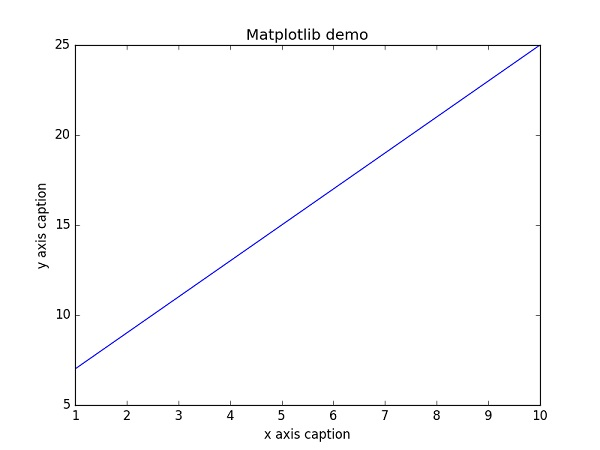Matplotlib Demo

`'-'`实线样式
`'--'`短横线样式
`'-.'`点划线样式
`':'`虚线样式
`'.'`点标记
`','`像素标记
`'o'`圆标记
`'v'`倒三角标记
`'^'`正三角标记
`'<'`左三角标记
`'>'`右三角标记
`'1'`下箭头标记
`'2'`上箭头标记
`'3'`左箭头标记
`'4'`右箭头标记
`'s'`正方形标记
`'p'`五边形标记
`'*'`星形标记
`'h'`六边形标记 1
`'H'`六边形标记 2
`'+'`加号标记
`'x'`X 标记
`'D'`菱形标记
`'d'`窄菱形标记
`''`竖直线标记
`'_'`水平线标记

`'b'`蓝色
`'g'`绿色
`'r'`红色
`'c'`青色
`'m'`品红色
`'y'`黄色
`'k'`黑色
`'w'`白色

### 示例

```import numpy as np
from matplotlib import pyplot as plt

x = np.arange(1,11) y =  2  * x +  5 plt.title("Matplotlib demo") plt.xlabel("x axis caption") plt.ylabel("y axis caption") plt.plot(x,y,"ob") plt.show()```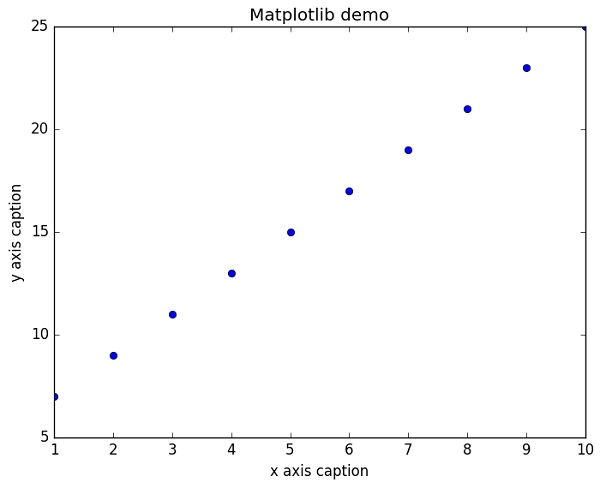Color Abbreviation

## 绘制正弦波

### 示例

```import numpy as np
import matplotlib.pyplot as plt
# 计算正弦曲线上点的 x 和 y 坐标x = np.arange(0,  3  * np.pi,  0.1) y = np.sin(x)plt.title("sine wave form")  # 使用 matplotlib 来绘制点plt.plot(x, y) plt.show()```Sine Wave

## `subplot()`

`subplot()`函数允许你在同一图中绘制不同的东西。 在下面的脚本中，绘制正弦余弦值。

### 示例

```import numpy as np
import matplotlib.pyplot as plt
# 计算正弦和余弦曲线上的点的 x 和 y 坐标 x = np.arange(0,  3  * np.pi,  0.1) y_sin = np.sin(x) y_cos = np.cos(x)  # 建立 subplot 网格，高为 2，宽为 1  # 激活第一个 subplotplt.subplot(2,  1,  1)  # 绘制第一个图像 plt.plot(x, y_sin) plt.title('Sine')  # 将第二个 subplot 激活，并绘制第二个图像plt.subplot(2,  1,  2) plt.plot(x, y_cos) plt.title('Cosine')  # 展示图像plt.show()```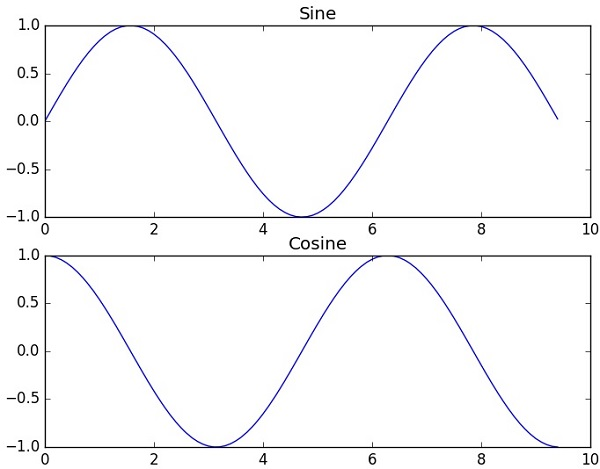Sub Plot

## `bar()`

`pyplot`子模块提供`bar()`函数来生成条形图。 以下示例生成两组`x``y`数组的条形图。

### 示例

```from matplotlib import pyplot as plt
x =  [5,8,10] y =  [12,16,6] x2 =  [6,9,11] y2 =  [6,15,7] plt.bar(x, y, align =  'center') plt.bar(x2, y2, color =  'g', align =  'center') plt.title('Bar graph') plt.ylabel('Y axis') plt.xlabel('X axis') plt.show()```

# NumPy - 使用 Matplotlib 绘制直方图

NumPy 有一个`numpy.histogram()`函数，它是数据的频率分布的图形表示。 水平尺寸相等的矩形对应于类间隔，称为`bin`，变量`height`对应于频率。

## `numpy.histogram()`

`numpy.histogram()`函数将输入数组和`bin`作为两个参数。 `bin`数组中的连续元素用作每个`bin`的边界。

```import numpy as np

a = np.array([22,87,5,43,56,73,55,54,11,20,51,5,79,31,27]) ]np.histogram(a,bins =  [0,20,40,60,80,100]) hist,bins = np.histogram(a,bins =  [0,20,40,60,80,100])  print hist
print bins```

`[3 4 5 2 1][0 20 40 60 80 100]`

## `plt()`

Matplotlib 可以将直方图的数字表示转换为图形。 `pyplot`子模块的`plt()`函数将包含数据和`bin`数组的数组作为参数，并转换为直方图。

```from matplotlib import pyplot as plt
import numpy as np

a = np.array([22,87,5,43,56,73,55,54,11,20,51,5,79,31,27]) plt.hist(a, bins =  [0,20,40,60,80,100]) plt.title("histogram") plt.show()```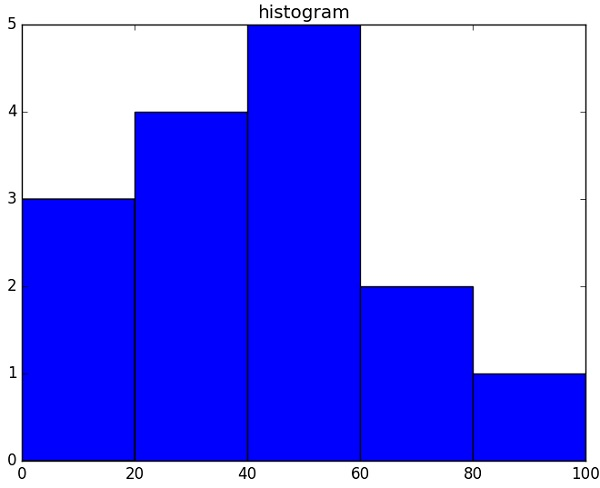Histogram Plot

# NumPy  - IO

`ndarray`对象可以保存到磁盘文件并从磁盘文件加载。 可用的 IO 功能有：

• `load()``save()`函数处理 numPy 二进制文件（带`npy`扩展名）

• `loadtxt()``savetxt()`函数处理正常的文本文件

NumPy 为`ndarray`对象引入了一个简单的文件格式。 这个`npy`文件在磁盘文件中，存储重建`ndarray`所需的数据、图形、`dtype`和其他信息，以便正确获取数组，即使该文件在具有不同架构的另一台机器上。

## `numpy.save()`

`numpy.save()`文件将输入数组存储在具有`npy`扩展名的磁盘文件中。

```import numpy as np
a = np.array([1,2,3,4,5]) np.save('outfile',a)```

```import numpy as np

`array([1, 2, 3, 4, 5])`

`save()``load()`函数接受一个附加的布尔参数`allow_pickles`。 Python 中的`pickle`用于在保存到磁盘文件或从磁盘文件读取之前，对对象进行序列化和反序列化。

## `savetxt()`

### 示例

```import numpy as np

a = np.array([1,2,3,4,5]) np.savetxt('out.txt',a) b = np.loadtxt('out.txt')  print b```

`[ 1.  2.  3.  4.  5.]`

`savetxt()``loadtxt()`数接受附加的可选参数，例如页首，页尾和分隔符。